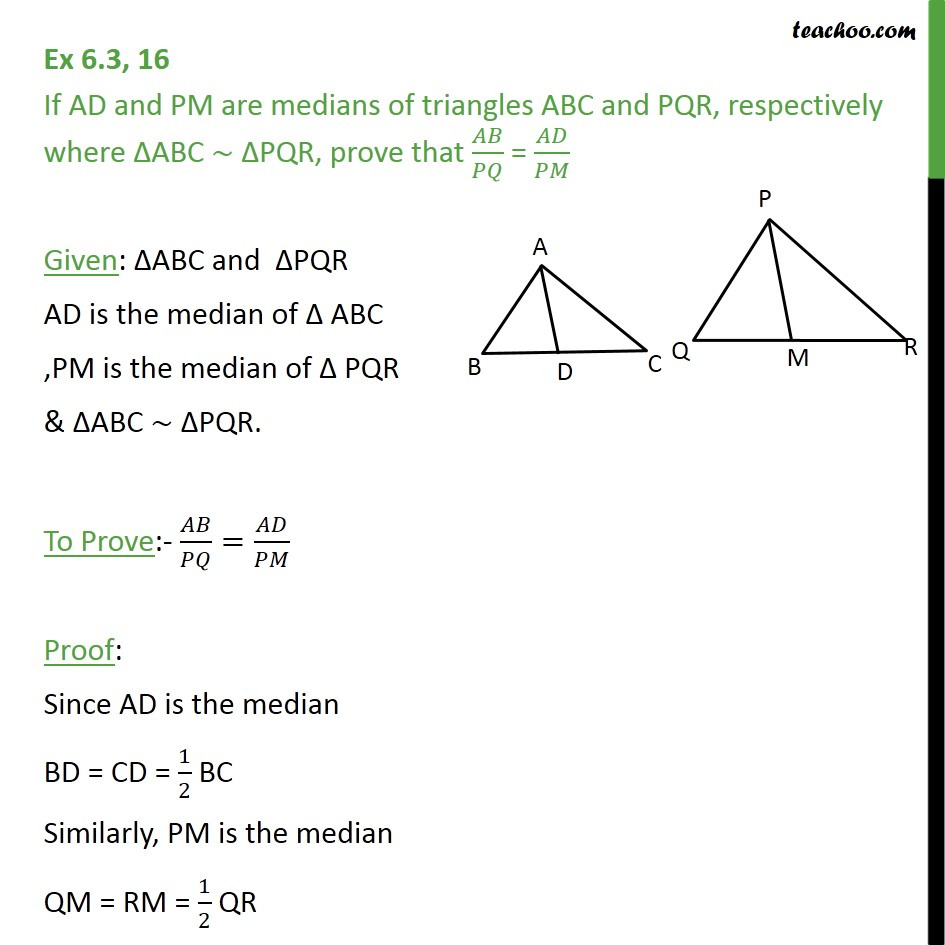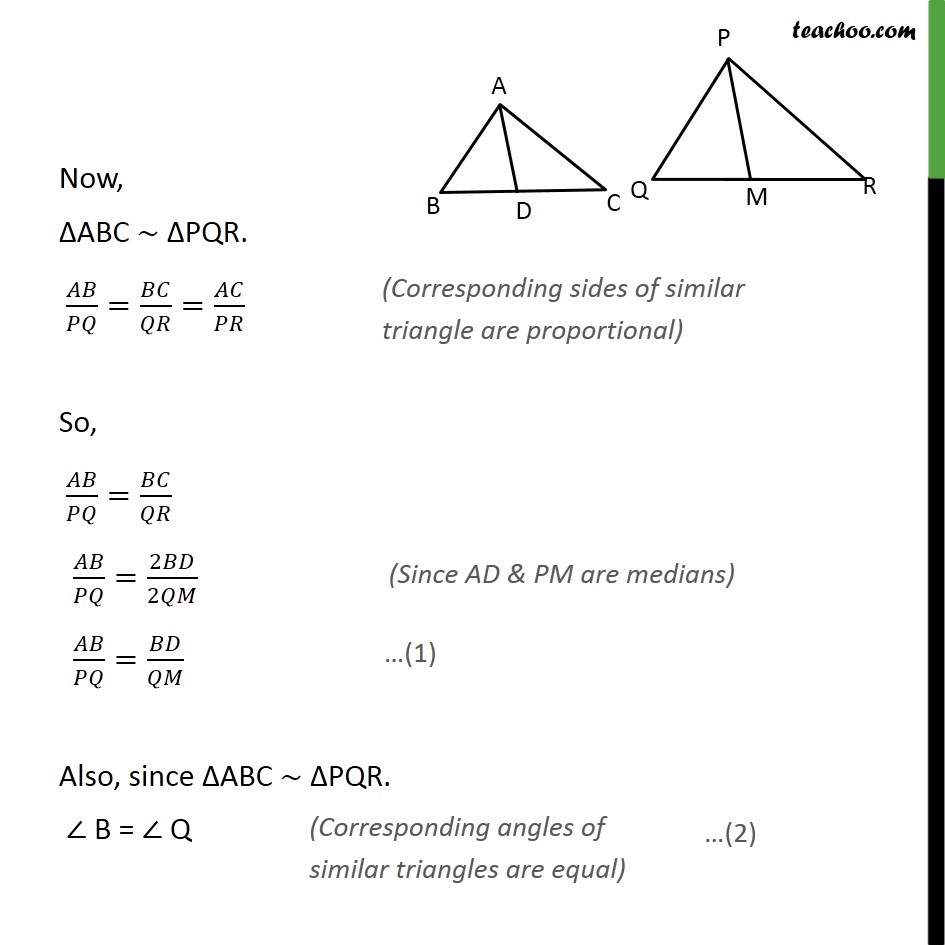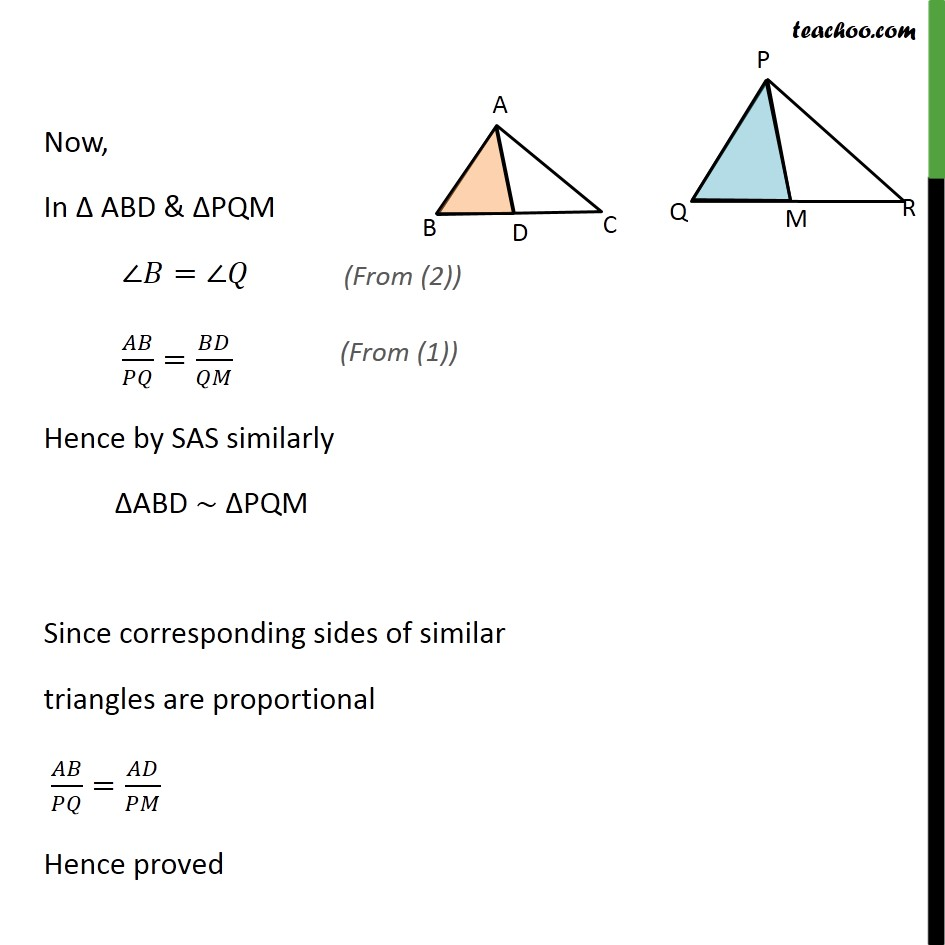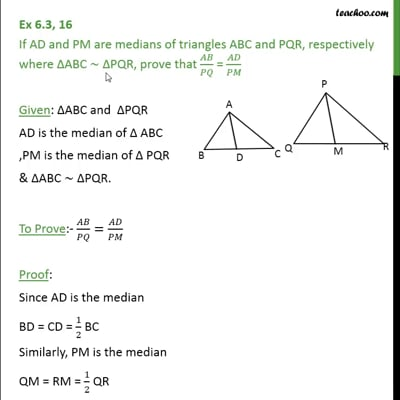Ex 6.3

Chapter 6 Class 10 Triangles (Term 1)
Serial order wiseThis video is only available for Teachoo black users

### Transcript

Ex 6.3, 16 If AD and PM are medians of triangles ABC and PQR, respectively where ABC PQR, prove that / = / Given: ABC and PQR AD is the median of ABC ,PM is the median of PQR & ABC PQR. To Prove:- / = / Proof: Since AD is the median BD = CD = 1/2 BC Similarly, PM is the median QM = RM = 1/2 QR Now, ABC PQR. / = / = / So, / = / / =2 /2 / = / Also, since ABC PQR. B = Q Now, In ABD & PQM = / = / Hence by SAS similarly ABD PQM Since corresponding sides of similar triangles are proportional / = / Hence proved